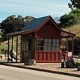# Gas station without pumps

## 2014 May 24

### Segmenting filtered signals

Last August, I posted on Segmenting noisy signals from nanopores, and in January I updated that with improved parameterization in More on segmenting noisy signals.  Last week, Jacob Schreiber (the programmer for the nanopore group) found some problems with the method. He had re-implemented the method in Cython (rather than Python, as I had prototyped it), and got the same results as me when applied to the same examples I had used. But when he applied it to some new data, he got serious oversegmentation, with hundred of segments chosen where only one should have been.

I tracked down the problem to a difference in how we were applying the method.  I was applying it to data that had been low-pass filtered at 5kHz, recorded at a sampling frequency of 100kHz, then downsampled to 10kHz.  He took the same data without downsampling, then further filtered it with a low-pass filter of 1kHz or 20kHz.  I confirmed that the same data would be correctly segmented with my downsampling and severely oversampled with his extra filtering.  He conjectured that the problem was with the sampling frequency and that the problem could be fixed by just changing the desired gain in log Prob based on sampling frequency.

His argument didn’t seem quite right, as the underlying math was not dependent on sampling frequency.  What it did assume was that the samples within a segment were independent Gaussian-distributed values (more correctly, that the difference between the samples and the model being fitted was Gaussian).  Putting in a low-pass filter removes the independence assumption.  Indeed, with a very low cutoff frequency you expect to see the values changing only slowly, so the segmenter would see it as highly desirable to chop the signal up into short segments, each of which has low variance.

I confirmed that I could get serious oversegmentation even with very low sampling frequency, if the filter cutoff was set much lower.  I got reasonable segmentation results with only minor variations across a wide range of sampling frequencies if the filter cutoff frequency was close to the Nyquist frequency (half the sampling frequency), but oversegmentation if I filtered to 0.1 Nyquist frequency.

There are two ways to address the problem:

• When filtering, always resample at twice the cutoff frequency.
• Adjust the thresholds used according to the cutoff frequency and sampling frequency.

Adjusting the thresholds is a cleaner approach, if it can be made to work.  Today I I tried filtering Gaussian noise with a 5th-order Bessel filter (implemented by scipy.signal.lfilter) and looking at the distribution of the log-odds that we threshold:$L_{i}=\log \frac{P_{\theta_{1}}(s:i) P_{\theta_{2}}(i:t)}{P_{\theta_{0}}(s:e)} = \log P_{\theta_{1}}(s:i) + \log P_{\theta_{2}}(i:t) - \log P_{\theta_{0}}(s:t)$,

where i is the breakpoint, θ1 and θ2 are the parameters for the two segments, and θ0 is the parameter setting for the whole segment.  For stepwise Gaussian models this simplifies to$L_{i} = (s-i)\ln \sigma_{1} + (i-t)\ln \sigma_{2} - (s-t)\ln \sigma_{0}$.   (To avoid the extra computation of taking square roots, the segmenter actually computes twice this, using variances instead of standard deviations.)

I found that the distributions were still very well fit by exponential distributions, but that the scaling factor changed with the cutoff frequency.   Furthermore, the log-odds seemed to scale linearly with the ratio of Nyquist frequency to cutoff frequency.  Reversing that scaling makes the curves superimpose nicely:If I scale the log-odds by filter cutoff frequency as a fraction of the Nyquist frequency, the distributions seem to be almost identical. Unfiltered Gaussians have slightly lower log-odds values. Filtering, then downsampling by averaging blocks of 10 samples has slightly higher log-odds, probably because the averaging does a little more low-pass filtering.

Each curve of the plot was computed from only 1000 sequences of 10000 samples, so the results are vary a lot in the tail, due to not having enough data to get the tail exactly.

Because the segmentation method is not very sensitive to the threshold set, simply scaling thresholds by Nyquist frequency/cutoff frequency should work well.   The default parameters had been set for the downsampled 5kHz, so to match the old results in signals  that aren’t downsampled, the thresholds should probably be scaled by about 1.1*Nyquist frequency/cutoff frequency.  (The analog filter cutoff frequency is probably in the metatdata in the data files, but the abf format is messy enough that I’ve not tried to extract it.  The lower cutoff frequency of the analog filter or a subsequent digital filter should be used.)

## 2 Comments »

1.[…] Segmenting filtered signals […]

Pingback by Segmenting noisy signals revisited | Gas station without pumps — 2014 June 17 @ 20:16

2.[…] up in this blog (with some improved parameterization): Segmenting noisy signals from nanopores, Segmenting filtered signals, and Segmenting noisy signals […]

Pingback by Two papers submitted | Gas station without pumps — 2014 August 19 @ 21:46

This site uses Akismet to reduce spam. Learn how your comment data is processed.